Next: The Three-Body Problem Up: Gravitational Potential Theory Previous: Perihelion Precession of Mercury

Exercises

1. Show that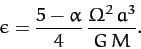for a self-gravitating, rotating spheroid of ellipticity, mass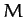, mean radius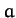, and angular velocity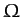whose mass density varies as(where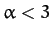). Demonstrate that the above formula matches the observed rotational flattening of the Earth whenand of Jupiter when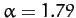.

2. The Moon's orbital period about the Earth is approximately 27.3 days, and is in the same direction as the Earth's axial rotation (whose period is 24 hours). Use this data to show that high tides at a given point on the Earth occur every 12 hours and 26 minutes.

3. Estimate the tidal elongation of the Moon due to the Earth.

4. Consider an artificial satellite in a circular orbit of radius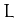about the Earth. Suppose that the normal to the plane of the orbit subtends an angle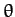with the Earth's axis of rotation. By approximating the orbiting satellite as a uniform ring, demonstrate that the Earth's oblateness causes the plane of the satellite's orbit to precess about the Earth's rotational axis at the rate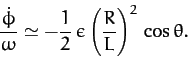Here,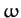is the satellite's orbital angular velocity,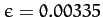the Earth's ellipticity, and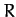the Earth's radius. Note that the Earth's axial moment of inertial is, whereis the mass of the Earth.

5. A sun-synchronous satellite is one which always passes over a given point on the Earth at the same local solar time. This is achieved by fixing the precession rate of the satellite's orbital plane such that it matches the rate at which the Sun appears to move against the background of the stars. What orbital altitude above the surface of the Earth would such a satellite need to have in order to fly over all latitudes betweenN andS? Is the direction of the satellite orbit in the same sense as the Earth's rotation (prograde), or the opposite sense (retrograde)?Next: The Three-Body Problem Up: Gravitational Potential Theory Previous: Perihelion Precession of Mercury
Richard Fitzpatrick 2011-03-31